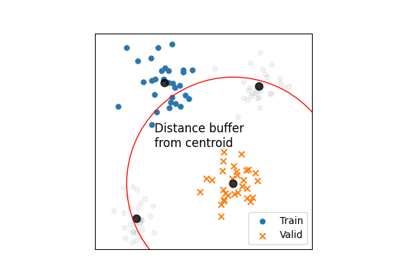# SpatialLeaveOneSubGroupOut¶

class `museotoolbox.cross_validation.``SpatialLeaveOneSubGroupOut`(distance_thresold, distance_matrix, distance_label, random_state=False, verbose=False)[source]

Generate a Cross-Validation with Spatial Leave-One-Out method.

Parameters
• distance_matrix (numpy.ndarray, shape [n_samples, n_samples]) – Array got from function `museotoolbox.vector_tools.get_distance_matrix()`

• distance_thresold (int.) – In pixels.

• distance_label (None or array.) – If array, got from function `museotoolbox.vector_tools.get_distance_matrix()`

• random_state (integer or None, optional (default=None)) – If int, random_state is the seed used by the random number generator; If None, the random number generator is created with `time.time()`.

• verbose (integer or False, optional (default=False)) – Controls the verbosity: the higher the value is, the more the messages are detailed.

`museotoolbox.processing.get_distance_matrix`

to get distance matrix and label.

Manage cross-validation methods to generate the duo valid/train samples.

Methods

 `__init__`(distance_thresold, distance_matrix, …) Manage cross-validation methods to generate the duo valid/train samples. `get_n_splits`([X, y, groups]) Returns the number of splitting iterations in the cross-validator. `save_to_vector`(vector, field[, group, …]) Save to vector files each fold from the cross-validation. `split`(X, y[, groups]) Split the vector/array according to y and groups.

## Examples using `museotoolbox.cross_validation.SpatialLeaveOneSubGroupOut`¶